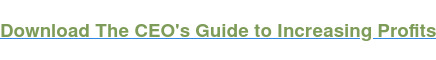# Cost Volume Profit Definition

A cost volume profit definition, defined also as the CVP model, is a financial model that shows how changes in sales volume, prices, and costs will affect profits. Use the CVP analysis for planning, making projections, and for decision-making purposes. A CVP model can be used to calculate a breakeven sales volume. CVP analysis can also be used to figure out the sales volume required to reach a certain target profit.## Cost Volume Profit Explanation

Cost volume profit, explained below, is one of the many ways to measure changes in the financial health of a company as it relates to sales. A CVP model is a simple financial model that assumes sales volume is the primary cost driver. In order to create a CVP model, you need certain data for the fiscal period in question. You need an estimate or figure for fixed costs, unit-level variable costs, and product/unit sales prices.

## Cost Volume Profit Examples

For example, let’s take a movie theater in reference to a simple cost volume profit analysis. The theater has quarterly fixed costs of \$30,000. These include utilities, salaries, and rent/mortgage, etc. The variable cost per movie ticket is \$2. This includes the cost of paper, printing, and the custodial services, etc. The price of a movie ticket is \$7.

Three variables:

1. Fixed costs of \$30,000
2. Variable costs of \$2
3. Sales price of \$7

Now, using this data, we can calculate the breakeven point for the theater. Once you have this data, calculating the breakeven point is easy. First, compute the contribution margin per ticket. The contribution margin is the sales price minus the unit-level variable costs. Then find out how many tickets the theater must sell in order to cover its fixed costs. To do this, divide fixed costs by the contribution margin per ticket.## Source

Hilton, Ronald W., Michael W. Maher, Frank H. Selto. “Cost Management Strategies for Business Decision”, Mcgraw-Hill Irwin, New York, NY, 2008.

1.